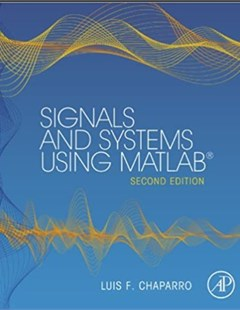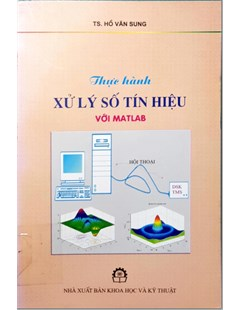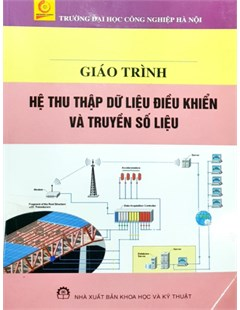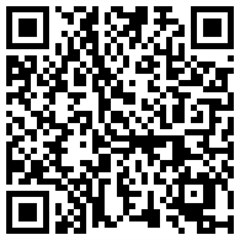Signals and systems using Matlab (Second edition)

This new textbook in signals and systems provides a pedagogically rich approach to what can commonly be a mathematically dry subject. With features like historical notes, highlighted common mistakes, and applications in controls, communications, and signal processing, Chaparro helps students appreciate the usefulness of the techniques described in the book. Each chapter contains a section with MatLab applications.

# Signals and systems using Matlab (Second edition)

2015

## Abstract

This new textbook in signals and systems provides a pedagogically rich approach to what can commonly be a mathematically dry subject. With features like historical notes, highlighted common mistakes, and applications in controls, communications, and signal processing, Chaparro helps students appreciate the usefulness of the techniques described in the book. Each chapter contains a section with MatLab applications.

The core of the material is presented in the second and third parts of the book. In the second part we cover the basics of continuous­time signals and systems and illustrate their applications, while in the third part we do similarly for discrete­time signals and system.

+ Chapters 1 and 2. In Chapter 1, we consider basic characteristics and processing of continuous­time signals, and provide the representation of signals using basic signals. Chapter 2 introduces the concept of systems, in particular of continuous­time systems. Linearity, time­invariance, causality, and stabil­ity are introduced in this chapter. Using linearity and time­invariance, the computation of the output of a continuous­time system using the convolution integral is introduced and illustrated.

+ Chapter 3 covers the basics of the Laplace transform and its application in the analysis of continuous­time signals and systems. It introduces the student to the concept of poles and zeros, damping and frequency, and their connec­tion with signals as functions of time.

+ Chapters 4 and 5. The Fourier series analysis of periodic signals, covered in Chapter 4, is extended to the analysis of aperiodic signals resulting in the Fourier transform of Chapter 5. The Fourier transform is useful in representing both periodic and aperiodic signals and for the frequency response of systems.

+ Chapters 6 and 7 present applications of the Laplace transform to control and of the Fourier transform to communications, and filtering. The intent of these chapters is to motivate interest in these areas. Chapter 6 illustrates the significance of the concepts of transfer function, response of systems, and stability in classical control. Relative to the first edition, we have added a section on the state­variable representation of systems—of great interest in modern control­and its connection to the Laplace transform. Chapter 7 illustrates the application of the Fourier analysis in communications and in analog filter­ing. Analytic as well as MATLAB examples illustrate different applications to control, communications, and filter design.

+ Chapter 8 presents the theory of sampling: the conditions under which the signal does not lose information in the sampling process and the recovery of the analog signal from the sampled signal as well as its application in digital communications.

## Citation

Luis F. Chaparro. Signals and systems using Matlab©. Elsevier, 2015.

## Collection

Ngành CÔNG NGHỆ KỸ THUẬT ĐIỆN TỬ - VIỄN THÔNG (7510302)

## Related documentSignals and systems using Matlab (Second edition) Thực hành xử lý số tín hiệu với Matlab Giáo trình hệ thu thập dữ liệu điều khiển và truyền số liệu

## QR code## Content

• Thứ Ba, 13:00 15/03/2022

### Tin tiêu điểm

Khai thác danh mục tạp chí mở Directory of Open Access Journals (DOAJ)

Thứ Sáu, 15:50 18/08/2023

Tài nguyên giáo dục mở Việt Nam (Vietnam Open Educational Resources – VOER)

Thứ Sáu, 19:07 02/12/2022

Tài nguyên truy cập mở Digital Commons Network

Thứ Sáu, 15:15 04/11/2022

### Các bài đã đăng

Reflect 4: Listening & Speaking (Student's Book)

Thứ Tư, 13:37 22/11/2023

Reflect 3: Reading & Writing (Teacher's guide)

Thứ Tư, 13:26 22/11/2023

Reflect 3: Listening & Speaking (Student's Book)

Thứ Tư, 13:14 22/11/2023

Giáo trình Vật lý 1

Thứ Ba, 12:56 14/11/2023

Giáo trình Quản trị chiến lược

Thứ Ba, 10:24 14/11/2023

Giáo trình Phiên dịch 1

Thứ Ba, 12:59 15/03/2022

Luyện dịch Anh - Việt, Việt - Anh

Thứ Ba, 12:39 15/03/2022

Dẫn luận ngôn ngữ học

Thứ Ba, 12:19 15/03/2022

Đường cách mệnh

Thứ Ba, 12:12 15/03/2022

Đại cương Ngôn ngữ học. (Tập 2): Ngữ dụng học

Thứ Bảy, 19:28 12/03/2022